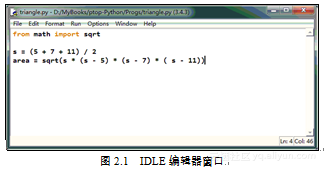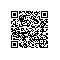# 《从问题到程序：用Python学编程和计算》——2.6　简单脚本程序

### 2.6　简单脚本程序

2.6.1　脚本的编辑和执行

IDLE的基本编辑功能与其他文本编辑器类似，特殊功能就是能自动维护Python程序的格式，用不同颜色突出显示程序里的各种成分，例如关键字、数值字面量、字符串等。用它编写Python程序非常方便。IDLE编辑器也支持各种常规的编辑操作，如通过键盘或鼠标移动编辑光标的位置，标记、复制、剪切和粘贴文本内容等。print函数的使用形式是：
print(表达式, 表达式, ……)


from math import sqrt

s = (5 + 7 + 11) / 2
area = sqrt(s * (s - 5) * (s - 7) * (s - 11))
print("Area of the triangle:", area)


>>> ===================== RESTART =======================
>>>
Area of triangle: 12.968712349342937

2.6.2　程序和输入

1）程序执行input(字符串)时，实参字符串将被输出到当时的交互执行环境，因此，这个字符串也就是一个要求使用者输入的提示，相当于Python解释器的提示符，通常称为提示符字符串或提示串，可以根据需要任意选择；
2）输出提示符后程序等待，直到用户输入了一些字符并按Enter键输入换行符；
3）input将用户在Enter键之前的输入做成一个字符串，作为函数的返回值。

from math import sqrt

a = float(input("Length of edge a: "))
b = float(input("Length of edge b: "))
c = float(input("Length of edge c: "))
s = (a + b + c) / 2
area = sqrt(s * (s - a) * (s - b) * (s - c))
print("Area of the triangle:", area)

>>>
Length of edge a: 5
Length of edge b: 7
Length of edge c: 11
Area of the triangle: 12.968712349342937

1）确定了三角形实例的一种描述方式：用三条边长描述三角形；
2）找到了一个通用的从三边长计算三角形面积的方法（直接来源于数学），基于这种方法写出了一段实现相应计算的程序（脚本）；
3）代码中包含了获取具体三角形信息（输入）和输出计算结果的部分。

>>>
Length of edge a: -2
Length of edge b: 6
Length of edge c: 10
Traceback (most recent call last):
File "D:/MyBooks/ptop-Python/Progs/triangle.py",
line 7, in <module>
area = sqrt(s * (s - a) * (s - b) * (s - c))
ValueError: math domain error

1）检查数据的情况，判断一些条件是否成立；
2）根据条件的成立与否，选择不同的操作或者操作序列。

Python程序里的注释用一个井号字符（#）开头，一直延伸到该字符所在行末尾。解释器处理中遇到 # 字符，就把它和直到换行的一段字符丢掉，不做任何处理。人们提倡把较长的注释写成独立的行，写在普通语句之后的短小注释也很常见。